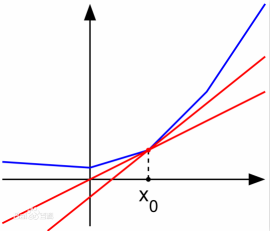# Logistic 回归的 Loss 函数为什么长成那个样子？

（以下是正常的版本。）

$n$ 是样本数量。

# 凸优化 及 梯度下降法

（顺便一说凸函数还有一个二阶条件：$\nabla^{2}f(x) \ge 0$ 。）（原图来自 百度百科：次导数

# 扩展：Softmax 模型的 Loss 函数—— Log-Sum-Exp 函数

（Affine composition 指的是：$f(x)$ 是凸的 $\Leftrightarrow f(Ax+b)$ 也是凸的，即对自变量做仿射变换不影响凸性。）

（对角优势矩阵：对矩阵 $A$ 的所有对角元，有 $A_{ii} \ge \sum_{i \ne j} |A_{ij}|$ 。）